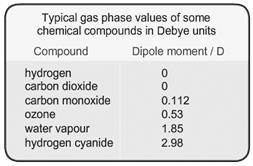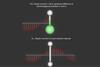Two balls separated by a spring will oscillate (harmonic) if stretched and released. The frequency of the vibrations depends on the strength of the spring ( ≡  bond strength) and the mass of the balls ( ≡   atoms).

The total energy of the vibration is given by the sum of the potential energies of the ‘compressed’ and ‘extended’ positions. This model suggests that the spring will vibrate at any energy dependent on the initial separation of the balls but, this is not true for molecules. The energy is quantised, which means that only certain energy levels are allowed according to the formula:

En = [n + ½]hν  where n = 0,1,2,3, etc.

In addition, molecules can only absorb (or emit) energy equal to the spacing between two levels and, for a harmonic oscillation, this can only occur between adjacent levels. However, bonds in real molecules do not vibrate harmonically.

When atoms approach each other closely, they exert a force of repulsion, and beyond a certain separation distance, a bond breaks. Quantisation produces unequal separations of energy levels which add complications to spectra. Finally, molecules will not absorb infrared radiation unless they possess a dipole, thus H2 is transparent to infrared whilst HCl absorbs.

Many molecules possess dipole moments due to non-uniform distributions of positive and negative charges on the various atoms

e.g.     Hδ+ - Clδ-

These (permanent) dipoles occur when two atoms in a molecule have substantially different electronegativities (see table below).Gas phase dipole moments

These values can be obtained from measurement of the dielectric constant. When the symmetry of a molecule cancels out, there is no net dipole moment and the value is therefore 0. The highest dipole moments are in the range of 10 to 11.

Information about the molecular geometry of a molecule can be deduced from the dipole moment. For example the data indicates that carbon dioxide (CO2) is a linear molecule but ozone (O3) is not.

• #### Ball and spring model

Simulation | Flash, Size 24.29 kb
• #### Dipole moment

Simulation | Flash, Size 6.32 kb

## Infrared (IR) spectroscopy

• 1
• 2
• 3Currently reading

• 4